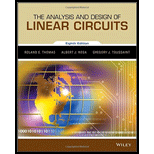## Browse All Chapters of This Textbook

### Book Details

The Analysis and Design of Linear Circuits, 8th Edition provides an introduction to the analysis, design, and evaluation of electric circuits, focusing on developing the learners design intuition. The text emphasizes the use of computers to assist in design and evaluation. Early introduction to circuit design motivates the student to create circuit solutions and optimize designs based on real-world constraints. This text is an unbound, three hole punched version.

## Sample Solutions for this Textbook

We offer sample solutions for ANALYSIS+DESIGN OF LINEAR CIRCUITS(LL) homework problems. See examples below:

## More Editions of This Book

Corresponding editions of this textbook are also available below:

Analysis And Design Linear Circuits
3rd Edition
ISBN: 9780471209294
Analysis And Design Of Linear Circuits
2nd Edition
ISBN: 9780471365921
The Analysis And Design Of Linear Circuits
5th Edition
ISBN: 9780471760955
EBK THE ANALYSIS AND DESIGN OF LINEAR C
8th Edition
ISBN: 9781119228226
ANALYSIS AND DESIGN OF LINEAR CIRCUTS
9th Edition
ISBN: 9781119593140
The Analysis and Design of Linear Circuits, Enhanced eText
9th Edition
ISBN: 9781119596196
Analysis And Design Of Linear Circuits
7th Edition
ISBN: 9781118065587